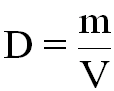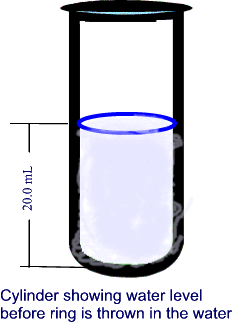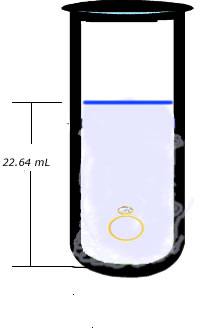#### The Density of Matter

Density by definition is the mass of a substance (how much matter it has) divided by its volume (how much space it occupies).where D =  density (kg/m3)

m =  mass (kg)

V =  volume (m3)

Density is a very important quantitative property of matter -- it helps identify a substances every substance has a specific density.  The density of a substance indicates how the particles of a substance are packed together win the substance.

The tighter the particles are packed, the higher the density of the substance.

The preferred SI (metric) unit for density is kg/m3

other units are also used:   g/L, g/dm3, g/cm3, g/mL

note that:               1 kg/m3 = 1 g/L = 1 g/dm3

1 g/cm3 = 1 g/mL = 1000 kg/m3

B.  Density Calculations:

1.  EQUIVALENT UNITS OF DENSITY

Conversions:

1000 kg/m3 = _________g/cm3

5.0 g/mL = __________kg/m3

25.67 g/cm3 = ________g/L

### INVESTIGATION:

Freddy wants to buy his sweetheart a gold friendship ring.  An acquaintance sells him a ring but the young is not sure if the ring is real gold or a fake.   Freddy remembers what he had learned in Mr. Lista's Science class, he goes in his science lab, puts the ring on a balance and finds out that the its mass is 29.8 g. He then borrows a graduated cylinder from Mr. Lista and fills it up to the 20 mL mark with water.  He then throws the ring in the graduated cylinder containing the water and notices that the level of the water now rises up to the 22.64 mL mark.

NOTE: Freddy used a method known as Measuring the Volume of an Object by the  Displacement of Water

The Bottom Line: Will Freddy's sweetheart be happy?  (i.e. is the ring pure gold?)

Let's help Freddy get to te bottom of this.

#### Solution:Note how the level of water rises once the ring is placed in the cylinder

### Conclusion:

Freddy checks the Density Table at the back of his Science textbook and sees that the density he calculated is actually that of the metal lead (11300 kg/m3). Therefore he concludes that  the ring is not gold.

Poor Freddy!!What is the actual density of Gold?